Dynamical systems (Part - 3) - Classical Mechanics, UGC - NET Physics

# Dynamical systems (Part - 3) - Classical Mechanics, UGC - NET Physics Notes | Study Physics for IIT JAM, UGC - NET, CSIR NET - Physics

## Document Description: Dynamical systems (Part - 3) - Classical Mechanics, UGC - NET Physics for Physics 2022 is part of Physics for IIT JAM, UGC - NET, CSIR NET preparation. The notes and questions for Dynamical systems (Part - 3) - Classical Mechanics, UGC - NET Physics have been prepared according to the Physics exam syllabus. Information about Dynamical systems (Part - 3) - Classical Mechanics, UGC - NET Physics covers topics like and Dynamical systems (Part - 3) - Classical Mechanics, UGC - NET Physics Example, for Physics 2022 Exam. Find important definitions, questions, notes, meanings, examples, exercises and tests below for Dynamical systems (Part - 3) - Classical Mechanics, UGC - NET Physics.

Introduction of Dynamical systems (Part - 3) - Classical Mechanics, UGC - NET Physics in English is available as part of our Physics for IIT JAM, UGC - NET, CSIR NET for Physics & Dynamical systems (Part - 3) - Classical Mechanics, UGC - NET Physics in Hindi for Physics for IIT JAM, UGC - NET, CSIR NET course. Download more important topics related with notes, lectures and mock test series for Physics Exam by signing up for free. Physics: Dynamical systems (Part - 3) - Classical Mechanics, UGC - NET Physics Notes | Study Physics for IIT JAM, UGC - NET, CSIR NET - Physics
 1 Crore+ students have signed up on EduRev. Have you?

Classi cation of critical points

In the previous idea we de ned the critical points and sketched how these points can be divided to stable and unstable points. We have seen that mathematical pendulum has two critical points, one is stable, the other is not. In the next example we have seen a system with two unstable critical points. The classi cation of critical points, however, is more subtle and we discuss all possibilities in this section.
Let us first recapitulate our goal. We study planar dynamical system described by equations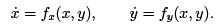We assume that we have found critical point of this system, i.e. point (xC, yC ) such that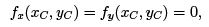and study the behaviour of the system near this critical point. We linearize the equations in the neighbourhood of critical point so that we obtain equations2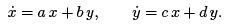This system can be written also in the matrix form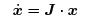where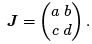Now we discuss several forms of matrix J and classify the critical points. Finally we will show how the analysis can be done for general matrix J .

Stable and unstable nodes, saddle points

Consider linear planar system of the form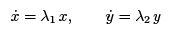(8.17)

which corresponds to matrix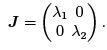(8.18)

System (8.17) can be easily solved. Equations for x and y are independent; we say that these equations are decoupled which means that equation for x_ does not contain y and vice versa.

Let us solve equation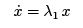first. In usual mathematical notation, this equation reads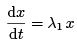which is separable dierential equation. We can rewrite it as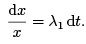This form of equation is called separated because the left hand side of the equations contains only x and the right hand side contains only time t. We can integrate the equation,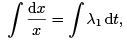to obtain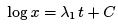where C is an integration constant. It is customary that if the logarithm appears in the solution, we write the constant as a logarithm as well3: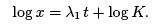Exponentiating the last equation we arrive at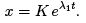By the same procedure we solve equation for y to get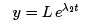where L is an integration constant again. Notice that, according to the solution, we have

x(0) = K and y(0) = L:

Hence, K and L are values of x and y at time t = 0, respectively. Therefore, we can write the solution of (8.17) in the form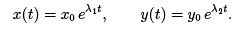(8.19)

Clearly, the only critical point of system (8.17) is (0; 0). Having derived solution of this system, we can analyze its behaviour near the critical point. Useful function to visualise properties of the system near critical point is StreamPlot which takes the vector eld and plots tra jectories. In the following example we choose λ= λ2 = 1.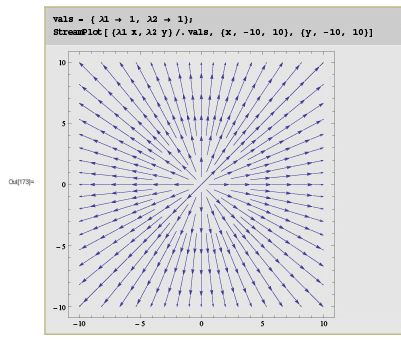In this figure we can see tra jectories (8.19) for initial points (x0, y0) chosen by Mathematica. Notice that we have inserted the right hand side of (8.17) as an argument of function StreamPlot. We can see that the trajectories are straight lines emanating from the origin (critical point) and tending to in nity exponentially.

What about other choices of λ1,2? It is clear that function eλt is increasing for λ > 0 and decreasing for λ < 0. We can conclude that qualitative behaviour of the system depends on signs of λ1,2 and four possibilities are shown in gure 8.8 which was created by following commands in Mathematica. We distinguish three cases.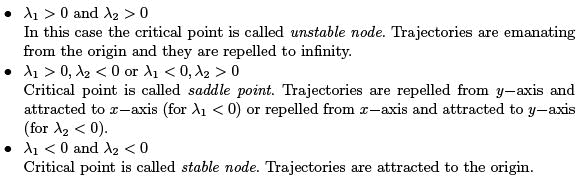In addition to this classi cation, critical points with distinct values λ 1 = λ 2 are called singular while critical points with the same values λ 1 = λ 2 are called degenerate.
Clearly, the saddle points cannot be singular.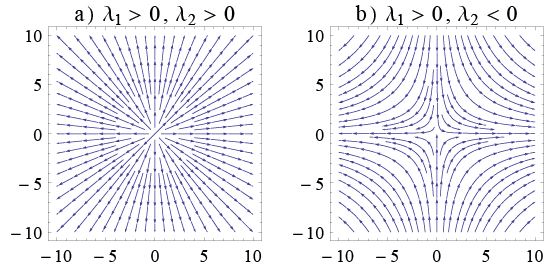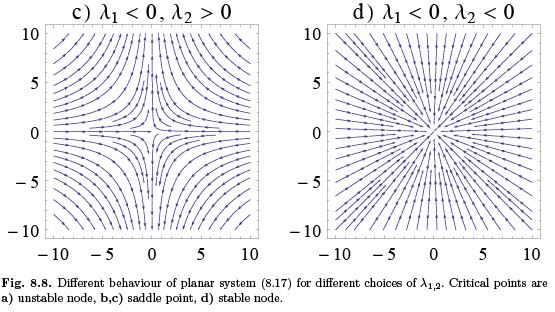Recall that planar dynamical system (8.17) can be represented by the matrix (8.18),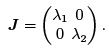From elementary linear algebra we know that with matrix J we can associate a set of eigenvalues λ defined by equation

J . e = λ e

where e is called an eigenvector. It is easy to show that the eigenvalues of matrix (8.18) are λand λ2 and corresponding eigenvectors are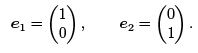In other words, vectors e1 and e2 satisfy equations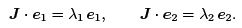We can see that tra jectories starting on lines determined by vectors ei, i = 1, 2, always remain in these lines. If the tra jectory is being repelled from the critical point along direction e, the line determined by vector e is called unstable manifold. If the tra jectory is attracted to the critical point along the vector e, the line determined by e is called stable manifold. For matrix (8.18), vectors e1 and e2 are always eigenvectors.
We can see that elies on the x axis and e2 lies on the y axis. Hence, the axes are stable or unstable manifolds of system (8.17), depending on the sign of λ1,2.

The classi cation introduced above can be reformulated in the following way. Let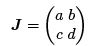be a matrix of general linear dynamical system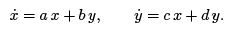If matrix J has two real eigenvalues λ1 and λ2, then critical point is stable/unstable node or a saddle point, depending on the signs of these eigenvalues.

We illustrate this classi cation on the example. Consider dynamical system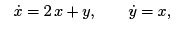(8.20)

with the matrix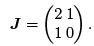This matrix is not of the form (8.18) but we can apply the second criterion. Eigenvalues and eigenvectors can be found in Mathematica using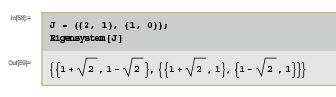which shows that eigenvalues are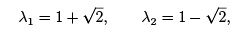and corresponding eigenvectors are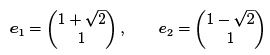Since λ1 > 0 and λ2 < 0, vector e1 de nes the stable manifold and ede nes unstable manifold. Since both eigenvalues have different signs, the critical point is a saddle point and it is regular. Phase tra jectories together with stable and unstable manifolds can be plotted by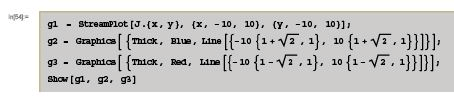The result is plotted in gure 8.9.

The document Dynamical systems (Part - 3) - Classical Mechanics, UGC - NET Physics Notes | Study Physics for IIT JAM, UGC - NET, CSIR NET - Physics is a part of the Physics Course Physics for IIT JAM, UGC - NET, CSIR NET.
All you need of Physics at this link: Physics

## Physics for IIT JAM, UGC - NET, CSIR NET

159 docs
 Use Code STAYHOME200 and get INR 200 additional OFF

## Physics for IIT JAM, UGC - NET, CSIR NET

159 docs

Track your progress, build streaks, highlight & save important lessons and more!

,

,

,

,

,

,

,

,

,

,

,

,

,

,

,

,

,

,

,

,

,

,

,

,

,

,

,

,

,

,

;